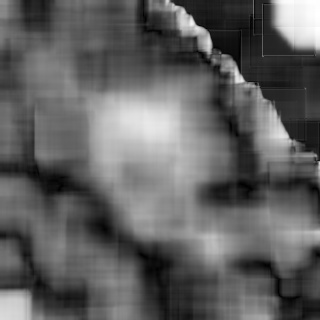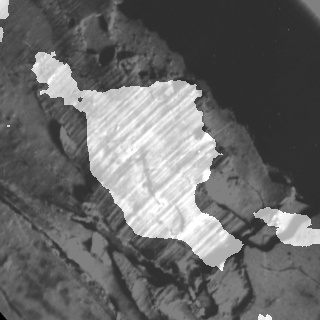OpenCV  4.0.0 Open Source Computer Vision
Anisotropic image segmentation by a gradient structure tensor

## Goal

In this tutorial you will learn:

• what the gradient structure tensor is
• how to estimate orientation and coherency of an anisotropic image by a gradient structure tensor
• how to segment an anisotropic image with a single local orientation by a gradient structure tensor

## Theory

Note
The explanation is based on the books ,  and . Good physical explanation of a gradient structure tensor is given in . Also, you can refer to a wikipedia page Structure tensor.

### What is the gradient structure tensor?

In mathematics, the gradient structure tensor (also referred to as the second-moment matrix, the second order moment tensor, the inertia tensor, etc.) is a matrix derived from the gradient of a function. It summarizes the predominant directions of the gradient in a specified neighborhood of a point, and the degree to which those directions are coherent (coherency). The gradient structure tensor is widely used in image processing and computer vision for 2D/3D image segmentation, motion detection, adaptive filtration, local image features detection, etc.

Important features of anisotropic images include orientation and coherency of a local anisotropy. In this paper we will show how to estimate orientation and coherency, and how to segment an anisotropic image with a single local orientation by a gradient structure tensor.

The gradient structure tensor of an image is a 2x2 symmetric matrix. Eigenvectors of the gradient structure tensor indicate local orientation, whereas eigenvalues give coherency (a measure of anisotropism).

The gradient structure tensor $$J$$ of an image $$Z$$ can be written as:

$J = \begin{bmatrix} J_{11} & J_{12} \\ J_{12} & J_{22} \end{bmatrix}$

where $$J_{11} = M[Z_{x}^{2}]$$, $$J_{22} = M[Z_{y}^{2}]$$, $$J_{12} = M[Z_{x}Z_{y}]$$ - components of the tensor, $$M[]$$ is a symbol of mathematical expectation (we can consider this operation as averaging in a window w), $$Z_{x}$$ and $$Z_{y}$$ are partial derivatives of an image $$Z$$ with respect to $$x$$ and $$y$$.

The eigenvalues of the tensor can be found in the below formula:

$\lambda_{1,2} = J_{11} + J_{22} \pm \sqrt{(J_{11} - J_{22})^{2} + 4J_{12}^{2}}$

where $$\lambda_1$$ - largest eigenvalue, $$\lambda_2$$ - smallest eigenvalue.

### How to estimate orientation and coherency of an anisotropic image by gradient structure tensor?

The orientation of an anisotropic image:

$\alpha = 0.5arctg\frac{2J_{12}}{J_{22} - J_{11}}$

Coherency:

$C = \frac{\lambda_1 - \lambda_2}{\lambda_1 + \lambda_2}$

The coherency ranges from 0 to 1. For ideal local orientation ( $$\lambda_2$$ = 0, $$\lambda_1$$ > 0) it is one, for an isotropic gray value structure ( $$\lambda_1$$ = $$\lambda_2$$ > 0) it is zero.

## Source code

You can find source code in the samples/cpp/tutorial_code/ImgProc/anisotropic_image_segmentation/anisotropic_image_segmentation.cpp of the OpenCV source code library.

#include <iostream>
using namespace cv;
using namespace std;
void calcGST(const Mat& inputImg, Mat& imgCoherencyOut, Mat& imgOrientationOut, int w);
int main()
{
int W = 52; // window size is WxW
double C_Thr = 0.43; // threshold for coherency
int LowThr = 35; // threshold1 for orientation, it ranges from 0 to 180
int HighThr = 57; // threshold2 for orientation, it ranges from 0 to 180
if (imgIn.empty()) //check whether the image is loaded or not
{
cout << "ERROR : Image cannot be loaded..!!" << endl;
return -1;
}
Mat imgCoherency, imgOrientation;
calcGST(imgIn, imgCoherency, imgOrientation, W);
Mat imgCoherencyBin;
imgCoherencyBin = imgCoherency > C_Thr;
Mat imgOrientationBin;
inRange(imgOrientation, Scalar(LowThr), Scalar(HighThr), imgOrientationBin);
Mat imgBin;
imgBin = imgCoherencyBin & imgOrientationBin;
normalize(imgCoherency, imgCoherency, 0, 255, NORM_MINMAX);
normalize(imgOrientation, imgOrientation, 0, 255, NORM_MINMAX);
imwrite("result.jpg", 0.5*(imgIn + imgBin));
imwrite("Coherency.jpg", imgCoherency);
imwrite("Orientation.jpg", imgOrientation);
return 0;
}
void calcGST(const Mat& inputImg, Mat& imgCoherencyOut, Mat& imgOrientationOut, int w)
{
Mat img;
inputImg.convertTo(img, CV_32F);
// GST components calculation (start)
// J = (J11 J12; J12 J22) - GST
Mat imgDiffX, imgDiffY, imgDiffXY;
Sobel(img, imgDiffX, CV_32F, 1, 0, 3);
Sobel(img, imgDiffY, CV_32F, 0, 1, 3);
multiply(imgDiffX, imgDiffY, imgDiffXY);
Mat imgDiffXX, imgDiffYY;
multiply(imgDiffX, imgDiffX, imgDiffXX);
multiply(imgDiffY, imgDiffY, imgDiffYY);
Mat J11, J22, J12; // J11, J22 and J12 are GST components
boxFilter(imgDiffXX, J11, CV_32F, Size(w, w));
boxFilter(imgDiffYY, J22, CV_32F, Size(w, w));
boxFilter(imgDiffXY, J12, CV_32F, Size(w, w));
// GST components calculation (stop)
// eigenvalue calculation (start)
// lambda1 = J11 + J22 + sqrt((J11-J22)^2 + 4*J12^2)
// lambda2 = J11 + J22 - sqrt((J11-J22)^2 + 4*J12^2)
Mat tmp1, tmp2, tmp3, tmp4;
tmp1 = J11 + J22;
tmp2 = J11 - J22;
multiply(tmp2, tmp2, tmp2);
multiply(J12, J12, tmp3);
sqrt(tmp2 + 4.0 * tmp3, tmp4);
Mat lambda1, lambda2;
lambda1 = tmp1 + tmp4; // biggest eigenvalue
lambda2 = tmp1 - tmp4; // smallest eigenvalue
// eigenvalue calculation (stop)
// Coherency calculation (start)
// Coherency = (lambda1 - lambda2)/(lambda1 + lambda2)) - measure of anisotropism
// Coherency is anisotropy degree (consistency of local orientation)
divide(lambda1 - lambda2, lambda1 + lambda2, imgCoherencyOut);
// Coherency calculation (stop)
// orientation angle calculation (start)
// tan(2*Alpha) = 2*J12/(J22 - J11)
// Alpha = 0.5 atan2(2*J12/(J22 - J11))
phase(J22 - J11, 2.0*J12, imgOrientationOut, true);
imgOrientationOut = 0.5*imgOrientationOut;
// orientation angle calculation (stop)
}

## Explanation

An anisotropic image segmentation algorithm consists of a gradient structure tensor calculation, an orientation calculation, a coherency calculation and an orientation and coherency thresholding:

Mat imgCoherency, imgOrientation;
calcGST(imgIn, imgCoherency, imgOrientation, W);
Mat imgCoherencyBin;
imgCoherencyBin = imgCoherency > C_Thr;
Mat imgOrientationBin;
inRange(imgOrientation, Scalar(LowThr), Scalar(HighThr), imgOrientationBin);
Mat imgBin;
imgBin = imgCoherencyBin & imgOrientationBin;

A function calcGST() calculates orientation and coherency by using a gradient structure tensor. An input parameter w defines a window size:

void calcGST(const Mat& inputImg, Mat& imgCoherencyOut, Mat& imgOrientationOut, int w)
{
Mat img;
inputImg.convertTo(img, CV_32F);
// GST components calculation (start)
// J = (J11 J12; J12 J22) - GST
Mat imgDiffX, imgDiffY, imgDiffXY;
Sobel(img, imgDiffX, CV_32F, 1, 0, 3);
Sobel(img, imgDiffY, CV_32F, 0, 1, 3);
multiply(imgDiffX, imgDiffY, imgDiffXY);
Mat imgDiffXX, imgDiffYY;
multiply(imgDiffX, imgDiffX, imgDiffXX);
multiply(imgDiffY, imgDiffY, imgDiffYY);
Mat J11, J22, J12; // J11, J22 and J12 are GST components
boxFilter(imgDiffXX, J11, CV_32F, Size(w, w));
boxFilter(imgDiffYY, J22, CV_32F, Size(w, w));
boxFilter(imgDiffXY, J12, CV_32F, Size(w, w));
// GST components calculation (stop)
// eigenvalue calculation (start)
// lambda1 = J11 + J22 + sqrt((J11-J22)^2 + 4*J12^2)
// lambda2 = J11 + J22 - sqrt((J11-J22)^2 + 4*J12^2)
Mat tmp1, tmp2, tmp3, tmp4;
tmp1 = J11 + J22;
tmp2 = J11 - J22;
multiply(tmp2, tmp2, tmp2);
multiply(J12, J12, tmp3);
sqrt(tmp2 + 4.0 * tmp3, tmp4);
Mat lambda1, lambda2;
lambda1 = tmp1 + tmp4; // biggest eigenvalue
lambda2 = tmp1 - tmp4; // smallest eigenvalue
// eigenvalue calculation (stop)
// Coherency calculation (start)
// Coherency = (lambda1 - lambda2)/(lambda1 + lambda2)) - measure of anisotropism
// Coherency is anisotropy degree (consistency of local orientation)
divide(lambda1 - lambda2, lambda1 + lambda2, imgCoherencyOut);
// Coherency calculation (stop)
// orientation angle calculation (start)
// tan(2*Alpha) = 2*J12/(J22 - J11)
// Alpha = 0.5 atan2(2*J12/(J22 - J11))
phase(J22 - J11, 2.0*J12, imgOrientationOut, true);
imgOrientationOut = 0.5*imgOrientationOut;
// orientation angle calculation (stop)
}

The below code applies a thresholds LowThr and HighThr to image orientation and a threshold C_Thr to image coherency calculated by the previous function. LowThr and HighThr define orientation range:

Mat imgCoherencyBin;
imgCoherencyBin = imgCoherency > C_Thr;
Mat imgOrientationBin;
inRange(imgOrientation, Scalar(LowThr), Scalar(HighThr), imgOrientationBin);

And finally we combine thresholding results:

Mat imgBin;
imgBin = imgCoherencyBin & imgOrientationBin;

## Result

Below you can see the real anisotropic image with single direction:Anisotropic image with the single direction

Below you can see the orientation and coherency of the anisotropic image:OrientationCoherency

Below you can see the segmentation result:Segmentation result

The result has been computed with w = 52, C_Thr = 0.43, LowThr = 35, HighThr = 57. We can see that the algorithm selected only the areas with one single direction.